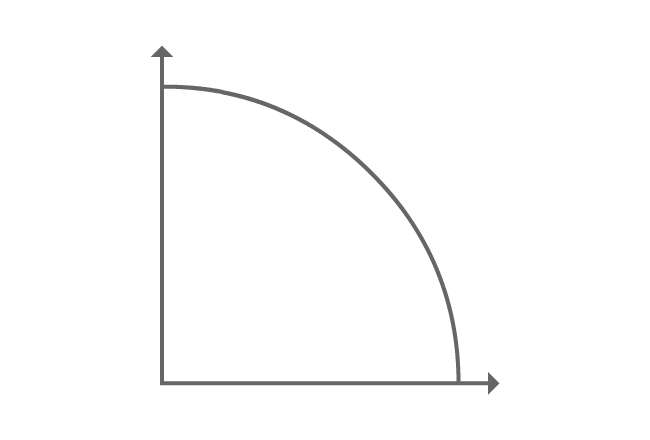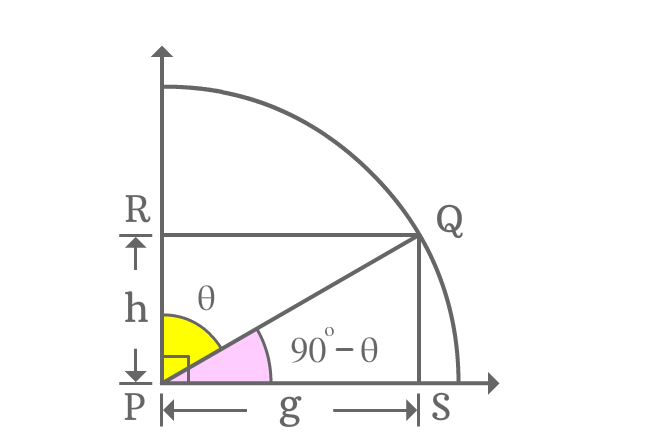# Cofunction identity of csc $($or$)$ cosec function

## Formula

Sexagesimal System
$\csc{(90^\circ-\theta)} \,\,$ (or) $\,\, \operatorname{cosec}{(90^\circ-\theta)} \,=\, \sec{\theta}$
$\csc{\Big(\dfrac{\pi}{2}-x\Big)} \,\,$ (or) $\,\, \operatorname{cosec}{\Big(\dfrac{\pi}{2}-x\Big)} \,=\, \sec{x}$

In cofunction identity formula, theta ($\theta$) is used to denote an angle in degrees and $x$ is used to denote an angle in radians.

Cosecant function contains an allied angle, which represent an angle in first quadrant. Hence, this trigonometric law is generally called as first quadrant’s allied angle identity of cosec function. In fact, the angles of both cosecant and secant functions are complementary angles in first quadrant. So, it is popularly called as cofunction identity of cosecant function.

### ProofA line segment is rotated to an angle and it is called as $\overline{PQ}$ mathematically.

From point $Q$, a perpendicular line is drawn to vertical ray and it intersects the ray at point $R$. Similarly, a perpendicular line is drawn to horizontal ray and it is intersected by the ray at point $S$.

Finally, it formed two right angled triangles geometrically, known as $\Delta QPR$ and $\Delta QPS$.

Take $\angle QPR = \theta$, therefore $\angle QPS = 90^\circ-\theta$.

Take $PS = g$, then $RQ = g$ and take $RP = h$, therefore $QS = h$ because of parallelism and equality of line segments.

#### Express cosec of allied angleIn first quadrant, the $\Delta QPS$ contains allied angle $90^\circ-\theta$ as angle. Write cosecant of allied angle as a ratio of lengths of the associated sides.

$\csc{(90^\circ-\theta)} = \dfrac{PQ}{QS}$

The length of opposite side is known but the length of the hypotenuse is unknown. It can be calculated on the basis of length of adjacent side by using Pythagorean Theorem.

$\implies \csc{(90^\circ-\theta)} = \dfrac{\sqrt{g^2+h^2}}{h}$

#### Find equivalent value of the fractionCosec of allied angle of first quadrant is written as a fraction and express the ratio as a trigonometric function by considering $\Delta QPR$.

$\dfrac{\sqrt{g^2+h^2}}{h} = \dfrac{PQ}{PR}$

The ratio of lengths of $\overline{PQ}$ to $\overline{PR}$ represents secant function in the $\Delta QPR$ and the angle of this triangle is theta. So, the value in fraction form is equal to secant of angle theta.

$\implies \dfrac{\sqrt{g^2+h^2}}{h} = \sec{\theta}$

#### Cofunction identity of cosec function

According to above two steps, it is derived that

$\csc{(90^\circ-\theta)}$ $=$ $\dfrac{\sqrt{g^2+h^2}}{h}$ $=$ $\sec{\theta}$

$\therefore \,\,\,\,\,\, \csc{(90^\circ-\theta)} \,=\, \sec{\theta}$

It can also be written as follows in trigonometric mathematics.

$\therefore \,\,\,\,\,\, \operatorname{cosec}{(90^\circ-\theta)} \,=\, \sec{\theta}$

It is proved in first quadrant that the value of cosecant of allied angle is equal to secant of angle. Therefore, the property of trigonometry is called as first quadrant’s allied angle of cosec function.

Due to the complementary angles of the cosecant and secant functions, the two functions are known as cofunctions and this identity is called as cofunction identity of cosecant function.

Latest Math Topics
Jun 26, 2023
Jun 23, 2023

Latest Math Problems
Jul 01, 2023
Jun 25, 2023
###### Math Questions

The math problems with solutions to learn how to solve a problem.

Learn solutions

Practice now

###### Math Videos

The math videos tutorials with visual graphics to learn every concept.

Watch now

###### Subscribe us

Get the latest math updates from the Math Doubts by subscribing us.